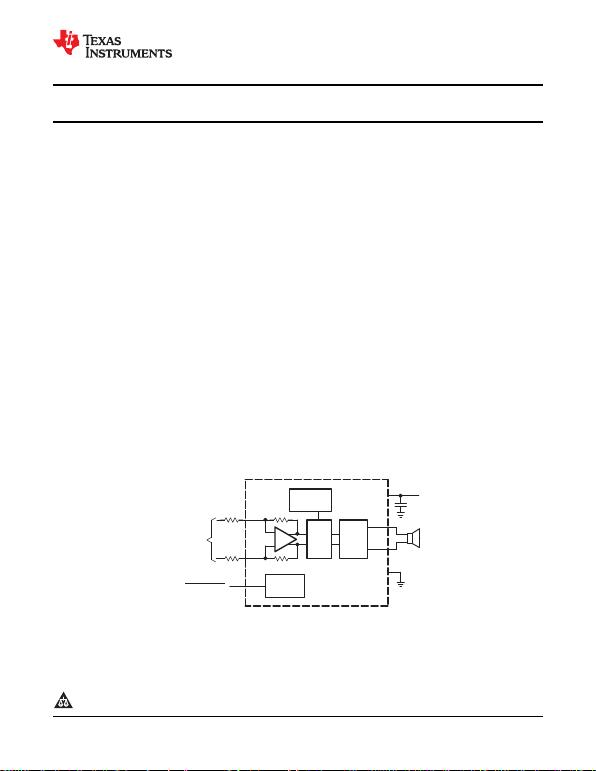_
+
IN–
IN+
PWM H–
Bridge
VO+
VO–
Internal
Oscillator CS
To Battery
VDD
GND
Bias
Circuitry
RI
RI
+
Differential
Input
TPA2005D1
SHUTDOWN
TPA2005D1-Q1
www.ti.com
SLOS474C AUGUST 2005REVISED MARCH 2010
1.4-W MONO FILTER-FREE CLASS-D AUDIO POWER AMPLIFIER
Check for Samples: TPA2005D1-Q1
1FEATURES
Qualified for Automotive Applications Space-Saving Packages
1.4 W Into 8 From a 5-V Supply at 3 mm x 3 mm QFN package (DRB)
THD = 10% (Typ) 3 mm x 5 mm MSOP PowerPAD™ Package
Maximum Battery Life and Minimum Heat (DGN)
Efficiency With an 8-Speaker: DESCRIPTION
84% at 400 mW The TPA2005D1 is a 1.4-W high-efficiency filter-free
79% at 100 mW class-D audio power amplifier in a QFN or MSOP
2.8-mA Quiescent Current package that requires only three external
0.5-mA Shutdown Current components.
Only Three External Components Features like 84% efficiency, –71-dB PSRR at
Optimized PWM Output Stage Eliminates 217 Hz, improved RF-rectification immunity, and
LC Output Filter 15-mm2total PCB area make the TPA2005D1 ideal
for cellular handsets. A fast start-up time of 9 ms with
Internally Generated 250-kHz Switching minimal pop makes the TPA2005D1 ideal for PDA
Frequency Eliminates Capacitor and applications.
Resistor In cellular handsets, the earpiece, speaker phone,
Improved PSRR (–71 dB at 217 Hz) and and melody ringer can each be driven by the
Wide Supply Voltage (2.5 V to 5.5 V) TPA2005D1. The device allows independent gain
Eliminates Need for a Voltage Regulator control by summing the signals from each function,
Fully Differential Design Reduces RF while minimizing noise to only 48 mVRMS.
Rectification and Eliminates Bypass
Capacitor
Improved CMRR Eliminates Two Input
Coupling Capacitors
APPLICATION CIRCUIT
1
Please be aware that an important notice concerning availability, standard warranty, and use in critical applications of Texas
Instruments semiconductor products and disclaimers thereto appears at the end of this data sheet.
PRODUCTION DATA information is current as of publication date. Copyright © 2005–2010, Texas Instruments Incorporated
Products conform to specifications per the terms of the Texas
Instruments standard warranty. Production processing does not
necessarily include testing of all parameters.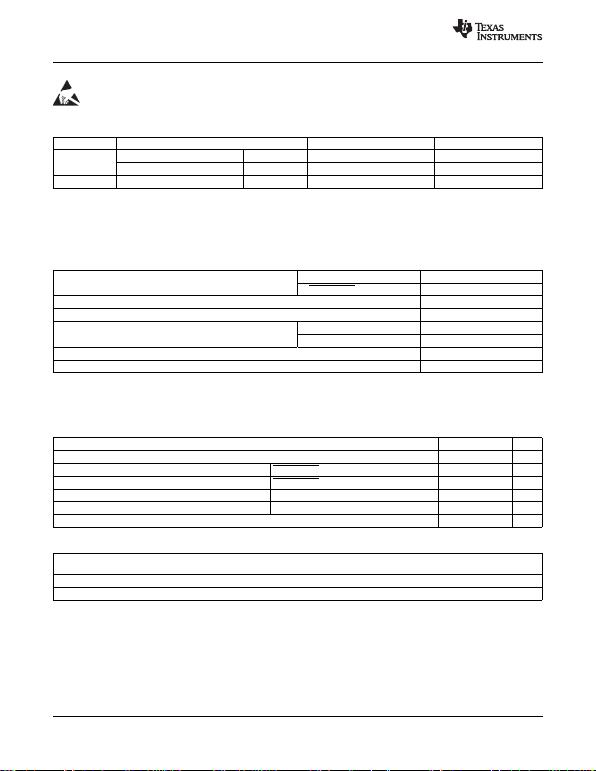TPA2005D1-Q1
SLOS474C AUGUST 2005REVISED MARCH 2010
www.ti.com
This integrated circuit can be damaged by ESD. Texas Instruments recommends that all integrated circuits be handled with
appropriate precautions. Failure to observe proper handling and installation procedures can cause damage.
ESD damage can range from subtle performance degradation to complete device failure. Precision integrated circuits may be more
susceptible to damage because very small parametric changes could cause the device not to meet its published specifications.
ORDERING INFORMATION(1)
TAPACKAGE(2) ORDERABLE PART NUMBER TOP-SIDE MARKING
QFN DRB Reel of 3000 TPA2005D1DRBRQ1 BIQ
–40°C to 85°C MSOP DGN Reel of 2500 TPA2005D1DGNRQ1 2005I
–40°C to 105°C MSOP DGN Reel of 2500 TPA2005D1TDGNRQ1 2005T
(1) For the most current package and ordering information, see the Package Option Addendum at the end of this document, or see the TI
web site at www.ti.com.
(2) Package drawings, thermal data, and symbolization are available at www.ti.com/packaging.
ABSOLUTE MAXIMUM RATINGS
over operating free-air temperature range unless otherwise noted(1)
In active mode –0.3 V to 6 V
VDD Supply voltage range(2) In SHUTDOWN mode –0.3 V to 7 V
VIInput voltage range –0.3 V to (VDD + 0.3 V)
Continuous total power dissipation See Dissipation Rating Table
Industrial range –40°C to 85°C
TAOperating free-air temperature range T-suffix range –40°C to 105°C
TJOperating junction temperature range –40°C to 150°C
Tstg Storage temperature range –65°C to 85°C
(1) Stresses beyond those listed under "absolute maximum ratings" may cause permanent damage to the device. These are stress ratings
only, and functional operation of the device at these or any other conditions beyond those indicated under "recommended operating
conditions" is not implied. Exposure to absolute-maximum-rated conditions for extended periods may affect device reliability.
(2) For the MSOP (DGN) package option, the maximum VDD should be limited to 5 V if short-circuit protection is desired.
RECOMMENDED OPERATING CONDITIONS MIN MAX UNIT
VDD Supply voltage 2.5 5.5 V
VIH High-level input voltage SHUTDOWN 2 VDD V
VIL Low-level input voltage SHUTDOWN 0 0.7 V
RIInput resistor Gain 20 V/V (26 dB) 15 k
VIC Common-mode input voltage range VDD = 2.5 V, 5.5 V, CMRR –49 dB 0.5 VDD 0.8 V
TAOperating free-air temperature –40 85 °C
DISSIPATION RATINGS
DERATING TA25°C TA= 70°C TA= 85°C
PACKAGE FACTOR POWER RATING POWER RATING POWER RATING
DRB 21.8 mW/°C 2.7 W 1.7 W 1.4 W
DGN 17.1 mW/°C 2.13 W 1.36 W 1.11 W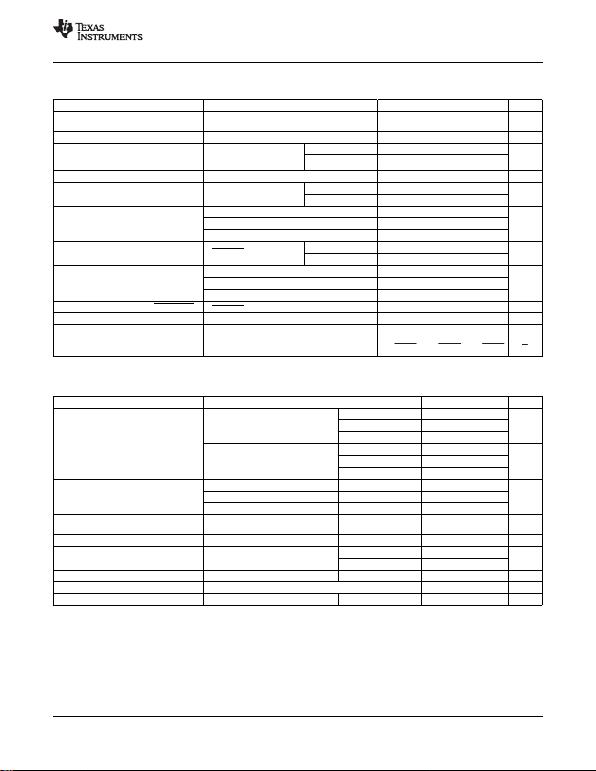V
V
2 142 kW
RI
2 158 kW
RI
2 150 kW
RI
TPA2005D1-Q1
www.ti.com
SLOS474C AUGUST 2005REVISED MARCH 2010
ELECTRICAL CHARACTERISTICS
TA= –40°C to 85°C (unless otherwise noted)
PARAMETER TEST CONDITIONS MIN TYP MAX UNIT
Output offset voltage (measured
|VOS| VI= 0 V, AV= 2 V/V, VDD = 2.5 V to 5.5 V 25 mV
differentially)
PSRR Power-supply rejection ratio VDD = 2.5 V to 5.5 V –75 –55 dB
VDD = 2.5 V to 5.5 V, TA= 25°C –68 –49
CMRR Common-mode rejection ratio VIC = VDD/2 to 0.5 V, dB
TA= –40°C to 85°C –35
VIC = VDD/2 to VDD 0.8 V
|IIH| High-level input current VDD = 5.5 V, VI= 5.8 V 50 mA
TA= –40°C to 85°C 4
|IIL| Low-level input current VDD = 5.5 V, VI= 0.3 V mA
TA= –40°C to 105°C 12
VDD = 5.5 V, no load 3.4 4.5
I(Q) Quiescent current VDD = 3.6 V, no load 2.8 mA
VDD = 2.5 V, no load 2.2 3.2
TA= –40°C to 85°C 0.5 2
V(SHUTDOWN) = 0.8 V,
I(SD) Shutdown current mA
VDD = 2.5 V to 5.5 V TA= –40°C to 105°C 2.5
VDD = 2.5 V 770
Static drain-source on-state
rDS(on) VDD = 3.6 V 590 m
resistance VDD = 5.5 V 500
Output impedance in SHUTDOWN V (SHUTDOWN) = 0.8 V >1 k
f(sw) Switching frequency VDD = 2.5 V to 5.5 V 200 250 300 kHz
Gain
OPERATING CHARACTERISTICS
TA= 25°C, Gain = 2 V/V, RL= 8 (unless otherwise noted)
PARAMETER TEST CONDITIONS MIN TYP MAX UNIT
VDD = 5 V 1.18
THD + N= 1%, f = 1 kHz, VDD = 3.6 V 0.58 W
RL= 8 VDD = 2.5 V 0.26
POOutput power VDD = 5 V 1.45
THD + N= 10%, f = 1 kHz, VDD = 3.6 V 0.75 W
RL= 8 VDD = 2.5 V 0.35
PO= 1 W, f = 1 kHz, RL= 8 VDD = 5 V 0.18%
THD+N Total harmonic distortion plus noise PO= 0.5 W, f = 1 kHz, RL= 8 VDD = 3.6 V 0.19%
PO= 200 mW, f = 1 kHz, RL= 8 VDD = 2.5 V 0.20%
f = 217 Hz, V(RIPPLE) = 200 mVpp,
kSVR Supply ripple rejection ratio VDD = 3.6 V 71 dB
Inputs ac-grounded with Ci= 2 mF
SNR Signal-to-noise ratio PO= 1 W, RL= 8 VDD = 5 V 97 dB
No weighting 48
VDD = 3.6 V, f = 20 Hz to 20 kHz,
VnOutput voltage noise mVRMS
Inputs ac-grounded with Ci= 2 mFA weighting 36
CMRR Common-mode rejection ratio VIC = 1 Vpp , f = 217 Hz VDD = 3.6 V 63 dB
ZIInput impedance 142 150 158 k
Start-up time from shutdown VDD = 3.6 V 9 ms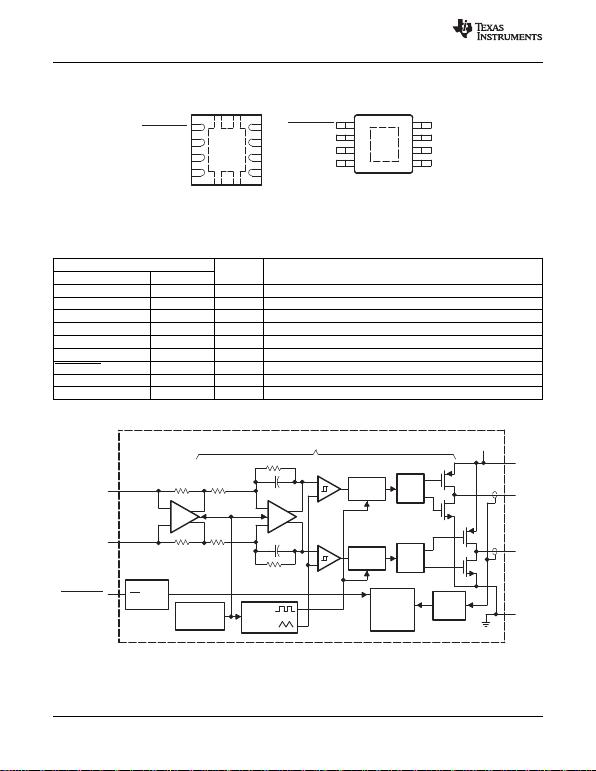8
SHUTDOWN
NC
IN+
IN−
VO−
GND
VDD
VO+
8-PIN QFN (DRB) PACKAGE
(TOP VIEW)
7
6
5
1
2
3
4
NC No internal connection
VO−
GND
VDD
VO+
8
7
6
5
1
2
3
4
SHUTDOWN
NC
IN+
IN−
8-PIN MSOP (DGN) PACKAGE
(TOP VIEW)
_
+_
+_
+_
+
150 k
150 k
_
+
_
+
Deglitch
Logic
Deglitch
Logic
Gate
Drive
Gate
Drive
VDD
Short
Circuit
Detect
Startup
& Thermal
Protection
Logic
Ramp
Generator
Biases
and
References
TTL
Input
Buffer
SD
Gain = 2 V/V B4, C4
VDD
A4VO
D4VO+
GND
D1
IN−
C1
IN+
A1
SHUTDOWN
A2, A3, B3, C2, C3, D2, D3
(terminal labels for MicroStar Junior package)
TPA2005D1-Q1
SLOS474C AUGUST 2005REVISED MARCH 2010
www.ti.com
PIN ASSIGNMENTS
A. The shaded terminals are used for electrical and thermal connections to the ground plane. All of the shaded terminals
must be electrically connected to ground. No connect (NC) terminals still need a pad and trace.
B. The thermal pad of the DRB and DGN packages must be electrically and thermally connected to a ground plane.
Terminal Functions
TERMINAL I/O DESCRIPTION
NAME NO.
IN– 4 I Negative differential input
IN+ 3 I Positive differential input
VDD 6 I Power supply
VO+ 5 O Positive BTL output
GND 7 I High-current ground
VO– 8 O Negative BTL output
SHUTDOWN 1 I Shutdown terminal (active low logic)
NC 2 No internal connection
Thermal Pad Must be soldered to a grounded pad on the PCB.
FUNCTIONAL BLOCK DIAGRAM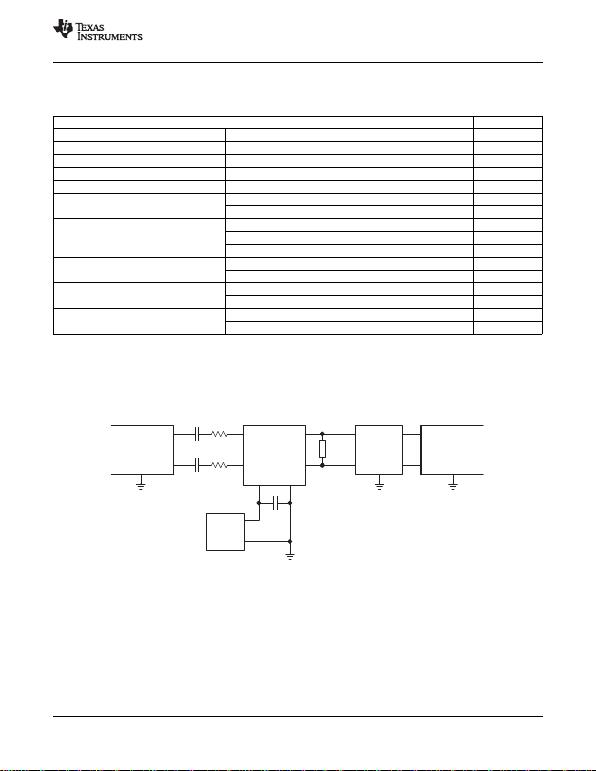TPA2005D1
IN+
IN−
OUT+
OUT−
VDD GND
CI
CI
RI
RI
Measurement
Output
+
1 µF
+
VDD
Low Pass
Filter
Measurement
Input
+
TPA2005D1-Q1
www.ti.com
SLOS474C AUGUST 2005REVISED MARCH 2010
TYPICAL CHARACTERISTICS
Table of Graphs
FIGURE
Efficiency vs Output power 1, 2
PDPower dissipation vs Output power 3
Supply current vs Output power 4, 5
I(Q) Quiescent current vs Supply voltage 6
I(SD) Shutdown current vs Shutdown voltage 7
vs Supply voltage 8
POOutput power vs Load resistance 9, 10
vs Output power 11, 12
THD+N Total harmonic distortion plus noise vs Frequency 13, 14, 15, 16
vs Common-mode input voltage 17
vs Frequency 18, 19, 20
kSVR Supply-voltage rejection ratio vs Common-mode input voltage 21
vs Time 22
GSM power-supply rejection vs Frequency 23
vs Frequency 24
CMRR Common-mode rejection ratio vs Common-mode input voltage 25
TEST SETUP FOR GRAPHS
A. CIwas shorted for any common-mode input voltage measurement.
B. A 33-mH inductor was placed in series with the load resistor to emulate a small speaker for efficiency measurements.
C. The 30-kHz low-pass filter is required, even if the analyzer has a low-pass filter. An RC filter (100 , 47 nF) is used
on each output for the data sheet graphs.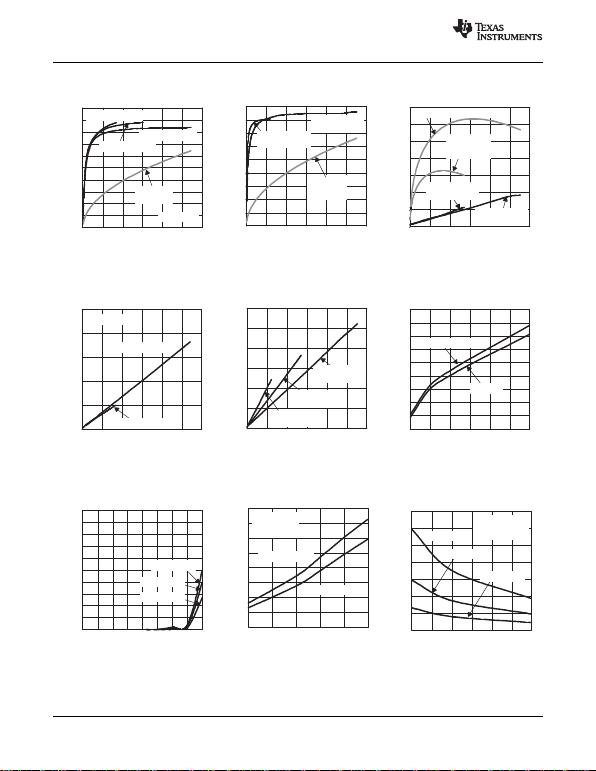0
10
20
30
40
50
60
70
80
90
0 0.2 0.4 0.6 0.8 1 1.2
VDD = 2.5 V,
RL= 8 , 33 µH
VDD = 5 V,
RL = 8 , 33 µH
Class-AB,
VDD = 5 V,
RL = 8
PO - Output Power - W
Efficiency - %
0
10
20
30
40
50
60
70
80
90
100
0 0.1 0.2 0.3 0.4 0.5 0.6
PO - Output Power - W
Efficiency - %
VDD = 3.6
RL = 32 , 33 µH
RL = 16 , 33 µH
RL = 8 , 33 µH
Class-AB,
RL = 8
0
50
100
150
200
250
300
0 0.2 0.4 0.6 0.8 1 1.2
PO - Output Power - W
VDD = 2.5 V,
RL = 8 , 33 µH
VDD = 3.6 V,
RL = 8 , 33 µH
VDD = 5 V,
RL = 8 , 33 µH
Supply Current - mA
2.5 3 3.5 4 4.5 5
VDD - Supply Voltage - V
- Output Power - WPO
RL = 8
f = 1 kHz
Gain = 2 V/V
THD+N = 1%
THD+N = 10%
0
0.2
0.4
0.6
0.8
1
1.2
1.4
1.6
0
0.1
0.2
0.3
0.4
0.5
0.6
0.7
0.8
0.9
1
0 0.1 0.2 0.3 0.4 0.5 0.6 0.7 0.8
VDD = 2.5 V
VDD = 3.6 V
VDD = 5 V
Shutdown Voltage - V
- Shutdown Current -
I(SD) Aµ
0
0.2
0.4
0.6
0.8
1
1.2
1.4
8 12 16 20 28
- Output Power - WPO
3224
f = 1 kHz
THD+N = 1%
Gain = 2 V/V
VDD = 2.5 V
VDD = 3.6 V
VDD = 5 V
TPA2005D1-Q1
SLOS474C AUGUST 2005REVISED MARCH 2010
www.ti.com
EFFICIENCY EFFICIENCY POWER DISSIPATION
vs vs vs
OUTPUT POWER OUTPUT POWER OUTPUT POWER
Figure 1. Figure 2. Figure 3.
SUPPLY CURRENT SUPPLY CURRENT QUIESCENT CURRENT
vs vs vs
OUTPUT POWER OUTPUT POWER SUPPLY VOLTAGE
Figure 4. Figure 5. Figure 6.
SHUTDOWN CURRENT OUTPUT POWER OUTPUT POWER
vs vs vs
SHUTDOWN VOLTAGE SUPPLY VOLTAGE LOAD RESISTANCE
Figure 7. Figure 8. Figure 9.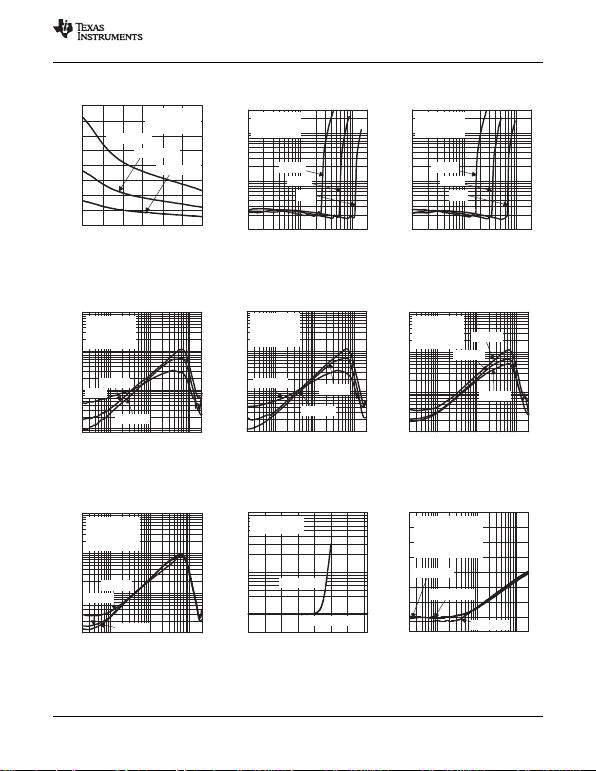0
0.2
0.4
0.6
0.8
1
1.2
1.4
1.6
8 12 16 20 24 28 32
VDD = 5 V
VDD = 3.6 V
VDD = 2.5 V
- Output Power - WPO
f = 1 kHz
THD+N = 10%
Gain = 2 V/V
0.1
30
0.2
0.5
1
2
5
10
20
0.01 20.1 1
PO − Output Power − W
THD+N − Total Harmonic Distortion + Noise − %
5 V
3.6 V
2.5 V
RL = 8 ,
f = 1 kHz,
Gain = 2 V/V
0.1
30
0.2
0.5
1
2
5
10
20
0.01 20.1 1
PO − Output Power − W
THD+N − Total Harmonic Distortion + Noise − %
5 V
3.6 V
2.5 V
RL = 16 ,
f = 1 kHz,
Gain = 2 V/V
0.008
10
0.02
0.05
0.1
0.2
0.5
1
2
5
20 100 1 k 20 k
f − Frequency − Hz
THD+N − Total Harmonic Distortion + Noise − %
50 mW
250 mW
1 W
VDD = 5 V
CI = 2 µF
RL = 8
Gain = 2 V/V
1
2
5
10
0.5
0.2
0.1
0.05
0.02
0.0120 100 1 k 20 k
f − Frequency − Hz
THD+N − Total Harmonic Distortion + Noise − %
VDD = 3.6 V
CI = 2 µF
RL = 8
Gain = 2 V/V
500 mW 25 mW
125 mW
10
5
2
1
0.5
0.2
0.1
0.05
0.02
0.0120 100 1 k 20 k
f − Frequency − Hz
THD+N − Total Harmonic Distortion + Noise − %
15 mW
VDD = 2.5 V
CI = 2 µF
RL = 8
Gain = 2 V/V
75 mW
200 mW
0.1
1
10
0 0.5 1 1.5 2 2.5 3 3.5
VDD = 2.5 V
VDD = 3.6 V
f = 1 kHz
PO = 200 mW
VIC - Common Mode Input Voltage - V
THD+N - Total Harmonic Distortion + Noise - %
−80
−70
−60
−50
−40
−30
−20
−10
0
20 100 1 k 20 k
f − Frequency − Hz
− Supply Voltage Rejection Ratio − dBkSVR
CI = 2 µF
RL = 8
Vp-p = 200 mV
Inputs ac-Grounded
Gain = 2 V/V
VDD = 5 V
VDD = 3.6 V
VDD =2. 5 V
VDD = 3.6 V
CI = 2 µF
RL = 16
Gain = 2 V/V
f − Frequency − Hz
THD+N − Total Harmonic Distortion + Noise − %
1
2
5
10
0.5
0.2
0.1
0.05
0.02
0.0120 100 1 k 20 k
15 mW
75 mW
200 mW
TPA2005D1-Q1
www.ti.com
SLOS474C AUGUST 2005REVISED MARCH 2010
OUTPUT POWER TOTAL HARMONIC DISTORTION + NOISE TOTAL HARMONIC DISTORTION + NOISE
vs vs vs
LOAD RESISTANCE OUTPUT POWER OUTPUT POWER
Figure 10. Figure 11. Figure 12.
TOTAL HARMONIC DISTORTION + NOISE TOTAL HARMONIC DISTORTION + NOISE TOTAL HARMONIC DISTORTION + NOISE
vs vs vs
FREQUENCY FREQUENCY FREQUENCY
Figure 13. Figure 14. Figure 15.
TOTAL HARMONIC DISTORTION + NOISE TOTAL HARMONIC DISTORTION + NOISE SUPPLY-VOLTAGE REJECTION RATIO
vs vs vs
FREQUENCY COMMON MODE INPUT VOLTAGE FREQUENCY
Figure 16. Figure 17. Figure 18.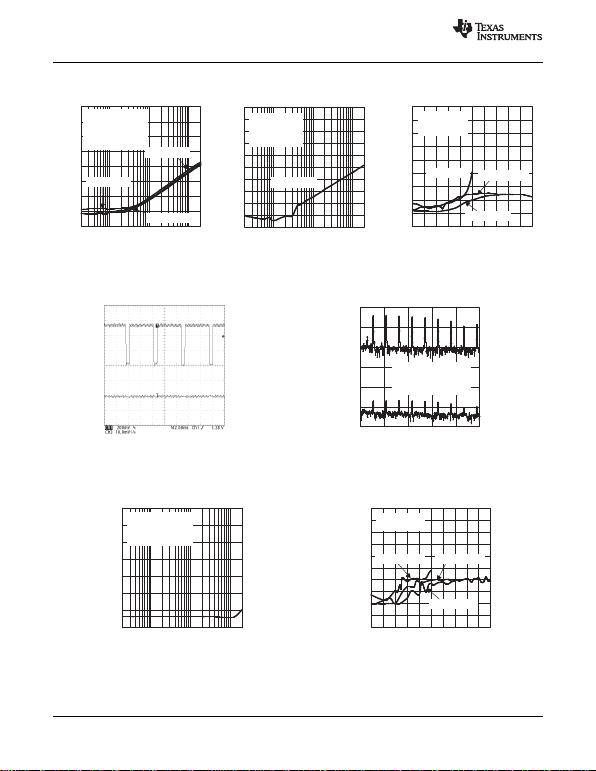−80
−70
−60
−50
−40
−30
−20
−10
0
f − Frequency − Hz
− Supply Voltage Rejection Ratio − dBkSVR
20 100 1 k 20 k
Gain = 5 V/V
CI = 2 µF
RL = 8
Vp-p = 200 mV
Inputs ac-Grounded
VDD = 5 V
VDD = 2. 5 V
VDD = 3.6 V
−100
−90
−80
−70
−60
−50
−40
−30
−20
−10
0
f − Frequency − Hz
− Supply Voltage Rejection Ratio − dBkSVR
VDD = 3.6 V
CI = 2 µF
RL = 8
Inputs Floating
Gain = 2 V/V
20 100 1 k 20 k
-90
-80
-70
-60
-50
-40
-30
-20
-10
0
0 0.5 1 1.5 2 2.5 3 3.5 4 4.5 5
VIC - Common Mode Input Voltage - V
f = 217 Hz
RL = 8
Gain = 2 V/V
VDD = 2.5 V
- Supply Voltage Rejection Ratio - dBkSVR
VDD = 3.6 V
VDD = 5 V
C1 - Duty
12.6%
C1 -
Frequency
216.7448 Hz
C1 - Amplitude
512 mV
C1 - High
3.544 V
Voltage - V
t - Time - ms
VDD
VOUT
-150
-100
-50
0 400 800 1200 1600 2000
-150
-100
-50
0
0
f - Frequency - Hz
- Output Voltage - dBVVO
- Supply Voltage - dBVVDD
VDD Shown in Figure 22
CI = 2 µF,
Inputs ac-grounded
Gain = 2V/V
−70
−60
−50
−40
−30
−20
−10
0
f − Frequency − Hz
CMRR − Common Mode Rejection Ratio − dB
20 100 1 k 20 k
VDD = 2.5 V to 5 V
VIC = 1 Vp−p
RL = 8
Gain = 2 V/V
-100
-90
-80
-70
-60
-50
-40
-30
-20
-10
0
0 0.5 1 1.5 2 2.5 3 3.5 4 4.5 5
RL = 8
Gain = 2 V/V
VIC - Common Mode Input Voltage - V
CMRR - Common Mode Rejection Ratio - dB
VDD = 5 V
VDD = 2.5 V VDD = 3.6 V
TPA2005D1-Q1
SLOS474C AUGUST 2005REVISED MARCH 2010
www.ti.com
SUPPLY-VOLTAGE REJECTION RATIO SUPPLY-VOLTAGE REJECTION RATIO SUPPLY-VOLTAGE REJECTION RATIO
vs vs vs
FREQUENCY FREQUENCY COMMON-MODE INPUT VOLTAGE
Figure 19. Figure 20. Figure 21.
GSM POWER-SUPPLY REJECTION GSM POWER-SUPPLY REJECTION
vs vs
TIME FREQUENCY
Figure 22. Figure 23.
COMMON-MODE REJECTION RATIO COMMON-MODE REJECTION RATIO
vs vs
FREQUENCY COMMON-MODE INPUT VOLTAGE
Figure 24. Figure 25.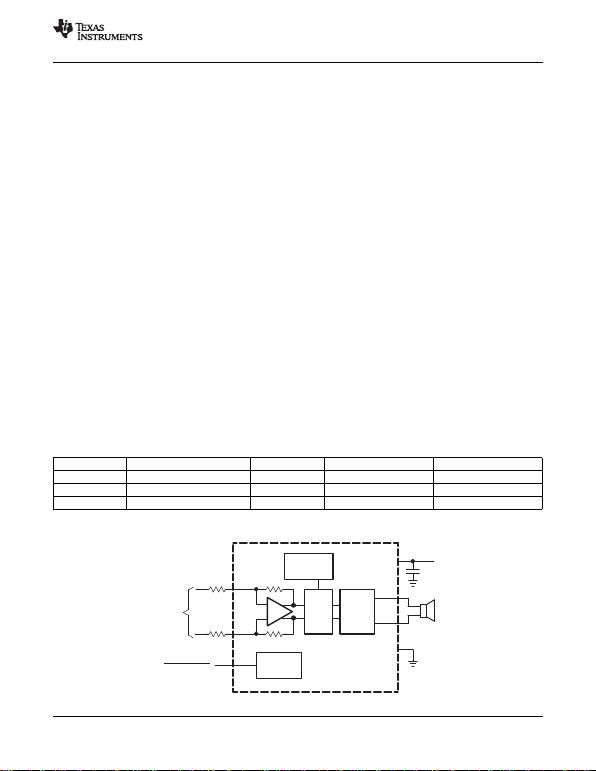_
+
IN–
IN+
PWM H–
Bridge
VO+
VO–
Internal
Oscillator CS
To Battery
VDD
GND
Bias
Circuitry
RI
RI
+
Differential
Input
TPA2005D1
Filter-Free Class D
SHUTDOWN
TPA2005D1-Q1
www.ti.com
SLOS474C AUGUST 2005REVISED MARCH 2010
APPLICATION INFORMATION
FULLY DIFFERENTIAL AMPLIFIER
The TPA2005D1 is a fully differential amplifier with differential inputs and outputs. The fully differential amplifier
consists of a differential amplifier and a common-mode amplifier. The differential amplifier ensures that the
amplifier outputs a differential voltage on the output that is equal to the differential input times the gain. The
common-mode feedback ensures that the common-mode voltage at the output is biased around VDD/2,
regardless of the common-mode voltage at the input. The fully differential TPA2005D1 can still be used with a
single-ended input; however, the TPA2005D1 should be used with differential inputs when in a noisy
environment, like a wireless handset, to ensure maximum noise rejection.
Input-coupling capacitors not required:
The fully differential amplifier allows the inputs to be biased at a voltage other than mid-supply. For
example, if a codec has a mid-supply lower than the mid-supply of the TPA2005D1, the common-mode
feedback circuit adjusts, and the TPA2005D1 outputs still is biased at mid-supply of the TPA2005D1. The
inputs of the TPA2005D1 can be biased from 0.5 V to VDD 0.8 V. If the inputs are biased outside of that
range, input-coupling capacitors are required.
Mid-supply bypass capacitor, C(BYPASS), not required:
The fully differential amplifier does not require a bypass capacitor. This is because any shift in the
mid-supply affects both positive and negative channels equally and cancels at the differential output.
Better RF immunity:
GSM handsets save power by turning on and shutting off the RF transmitter at a rate of 217 Hz. The
transmitted signal is picked up on input and output traces. The fully differential amplifier cancels the signal
much better than the typical audio amplifier.
COMPONENT SELECTION
Figure 26 shows the TPA2005D1 typical schematic with differential inputs, and Figure 27 shows the TPA2005D1
with differential inputs and input capacitors, and Figure 28 shows the TPA2005D1 with single-ended inputs.
Differential inputs should be used whenever possible, because the single-ended inputs are much more
susceptible to noise.
Table 1. Typical Component Values
REF DES VALUE EIA SIZE MANUFACTURER PART NUMBER
RI150 k0.5%) 0402 Panasonic ERJ2RHD154V
CS1mF (+22%, –80%) 0402 Murata GRP155F50J105Z
CI(1) 3.3 nF 10%) 0201 Murata GRP033B10J332K
(1) CIis needed only for single-ended input or if VICM is not between 0.5 V and VDD 0.8 V. CI= 3.3 nF (with RI= 150 k) gives a
high-pass corner frequency of 321 Hz.
Figure 26. Typical TPA2005D1 Application Schematic With Differential Input for a Wireless Phone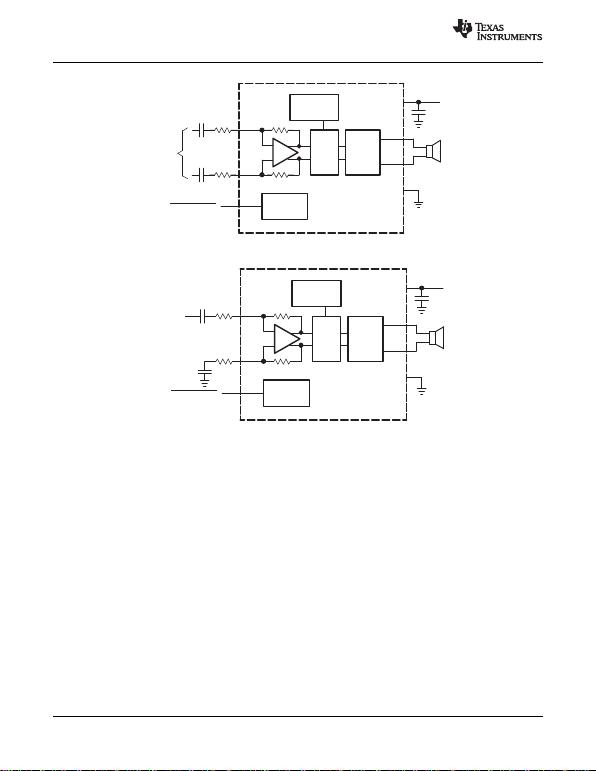_
+
IN–
IN+
PWM H–
Bridge
VO+
VO–
Internal
Oscillator CS
To Battery
VDD
GND
Bias
Circuitry
RI
RI
Differential
Input
TPA2005D1
Filter-Free Class D
SHUTDOWN
CI
CI
_
+
IN–
IN+
PWM H–
Bridge
VO+
VO–
Internal
Oscillator CS
To Battery
VDD
GND
Bias
Circuitry
RI
RI
Single-ended
Input
TPA2005D1
Filter-Free Class D
SHUTDOWN
CI
CI
TPA2005D1-Q1
SLOS474C AUGUST 2005REVISED MARCH 2010
www.ti.com
Figure 27. TPA2005D1 Application Schematic With Differential Input and Input Capacitors
Figure 28. TPA2005D1 Application Schematic With Single-Ended Input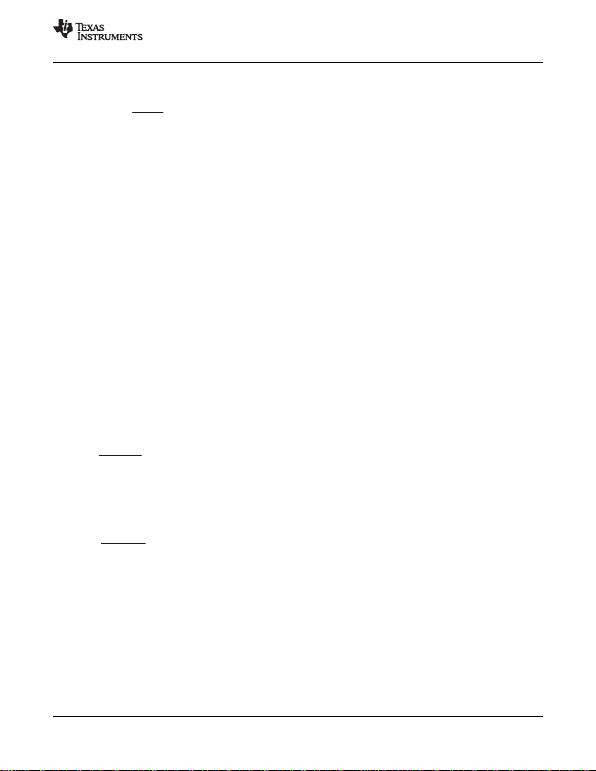Gain +2 150 kW
RI
fc+1
ǒ2pRICIǓ
CI+1
ǒ2pRIfcǓ
TPA2005D1-Q1
www.ti.com
SLOS474C AUGUST 2005REVISED MARCH 2010
Input Resistors (RI)
The input resistors (RI) set the gain of the amplifier according to equation Equation 1.
(1)
Resistor matching is very important in fully differential amplifiers. The balance of the output on the
reference voltage depends on matched ratios of the resistors. CMRR, PSRR, and cancellation of the second
harmonic distortion diminish if resistor mismatch occurs. Therefore, it is recommended to use 1% tolerance
resistors, or better, to keep the performance optimized. Matching is more important than overall tolerance.
Resistor arrays with 1% matching can be used with a tolerance greater than 1%.
Place the input resistors very close to the TPA2005D1 to limit noise injection on the high-impedance nodes.
For optimal performance, the gain should be set to 2 V/V or lower. Lower gain allows the TPA2005D1 to operate
at its best and keeps a high voltage at the input, making the inputs less susceptible to noise.
Decoupling Capacitor (CS)
The TPA2005D1 is a high-performance class-D audio amplifier that requires adequate power-supply decoupling
to ensure the efficiency is high and total harmonic distortion (THD) is low. For higher frequency transients,
spikes, or digital hash on the line, a good low equivalent-series-resistance (ESR) ceramic capacitor, typically
1mF, placed as close as possible to the device VDD lead, works best. Placing this decoupling capacitor close to
the TPA2005D1 is very important for the efficiency of the class-D amplifier, because any resistance or
inductance in the trace between the device and the capacitor can cause a loss in efficiency. For filtering
lower-frequency noise signals, a 10-mF, or greater, capacitor placed near the audio power amplifier also helps,
but it is not required in most applications because of the high PSRR of this device.
Input Capacitors (CI)
The TPA2005D1 does not require input coupling capacitors if the design uses a differential source that is biased
from 0.5 V to VDD 0.8 V (shown in Figure 26). If the input signal is not biased within the recommended
common-mode input range, if needing to use the input as a high pass filter (shown in Figure 27), or if using a
single-ended source (shown in Figure 28), input coupling capacitors are required.
The input capacitors and input resistors form a high-pass filter with the corner frequency, fc, determined in
Equation 2.
(2)
The value of the input capacitor is important to consider, as it directly affects the bass (low frequency)
performance of the circuit. Speakers in wireless phones usually cannot respond well to low frequencies, so the
corner frequency can be set to block low frequencies in this application.
Equation 3 is reconfigured to solve for the input coupling capacitance.
(3)
If the corner frequency is within the audio band, the capacitors should have a tolerance of ±10% or better,
because any mismatch in capacitance causes an impedance mismatch at the corner frequency and below.
For a flat low-frequency response, use large input coupling capacitors (1 mF). However, in a GSM phone the
ground signal is fluctuating at 217 Hz, but the signal from the codec does not have the same 217-Hz fluctuation.
The difference between the two signals is amplified, sent to the speaker, and heard as a 217-Hz hum.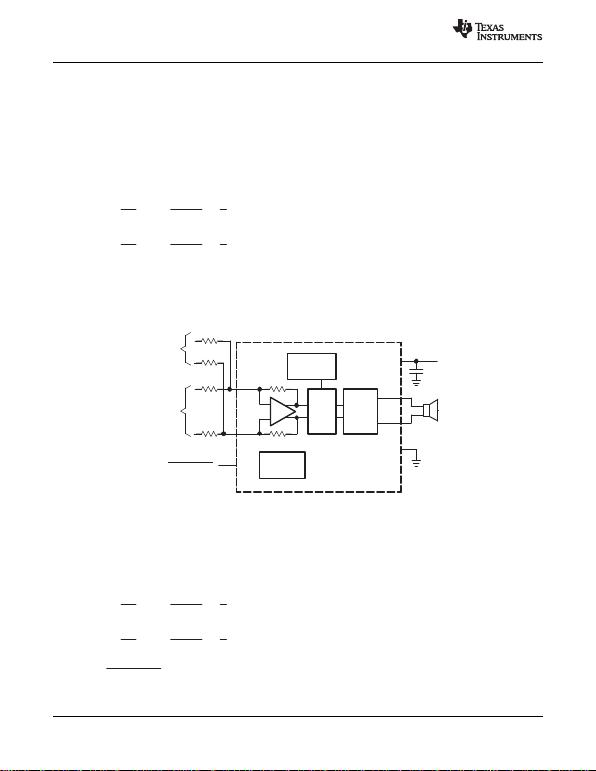Gain 1 +VO
VI1 +2 150 kW
RI1 ǒV
VǓ
Gain 2 +VO
VI2 +2 150 kW
RI2 ǒV
VǓ
_
+
IN–
IN+
PWM H–
Bridge
VO+
VO–
Internal
Oscillator CS
To Battery
VDD
GND
Bias
Circuitry
RI2
RI2
+
Differential
Input 1
SHUTDOWN
RI1
RI1
+
Differential
Input 2
Filter-Free Class D
Gain 1 +VO
VI1 +2 150 kW
RI1 ǒV
VǓ
Gain 2 +VO
VI2 +2 150 kW
RI2 ǒV
VǓ
CI2 +1
ǒ2pRI2 fc2Ǔ
TPA2005D1-Q1
SLOS474C AUGUST 2005REVISED MARCH 2010
www.ti.com
SUMMING INPUT SIGNALS WITH THE TPA2005D1
Most wireless phones or PDAs need to sum signals at the audio power amplifier or just have two signal sources
that need separate gain. The TPA2005D1 makes it easy to sum signals or use separate signal sources with
different gains. Many phones now use the same speaker for the earpiece and ringer, where the wireless phone
would require a much lower gain for the phone earpiece than for the ringer. PDAs and phones that have stereo
headphones require summing of the right and left channels to output the stereo signal to the mono speaker.
Summing Two Differential Input Signals
Two extra resistors are needed for summing differential signals (a total of 5 components). The gain for each input
source can be set independently (see Equation 4 and Equation 5 and Figure 29).
(4)
(5)
If summing left and right inputs with a gain of 1 V/V, use RI1= RI2= 300 k.
If summing a ring tone and a phone signal, set the ring-tone gain to gain 2 = 2 V/V, and the phone gain to
gain 1 = 0.1 V/V. The resistor values are:
RI1 =3Mand RI2 = 150 k.
Figure 29. Application Schematic With TPA2005D1 Summing Two Differential Inputs
Summing a Differential Input Signal and a Single-Ended Input Signal
Figure 30 shows how to sum a differential input signal and a single-ended input signal. Ground noise can couple
in through IN+ with this method. It is better to use differential inputs. The corner frequency of the single-ended
input is set by CI2, shown in Equation 8. To ensure that each input is balanced, the single-ended input must be
driven by a low-impedance source even if the input is not in use.
(6)
(7)
(8)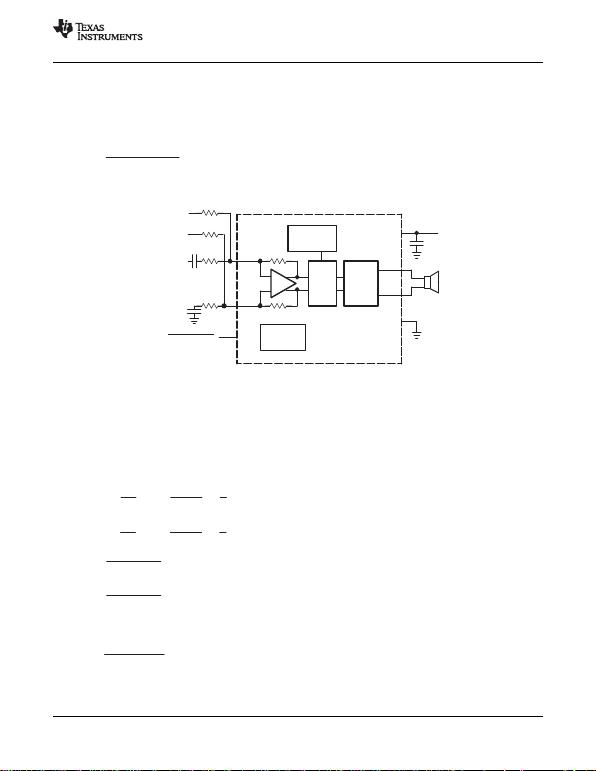CI2 u1
ǒ2p150kW20HzǓ
CI2 u53pF
_
+
IN–
IN+
PWM H–
Bridge
VO+
VO–
Internal
Oscillator CS
To Battery
VDD
GND
Bias
Circuitry
RI2
RI2
Differential
Input 1
Filter-Free Class D
SHUTDOWN
RI1
RI1
Single-Ended
Input 2
CI2
CI2
Gain 1 +VO
VI1 +2 150 kW
RI1 ǒV
VǓ
Gain 2 +VO
VI2 +2 150 kW
RI2 ǒV
VǓ
CI1 +1
ǒ2pRI1 fc1Ǔ
CI2 +1
ǒ2pRI2 fc2Ǔ
CP+CI1 )CI2
RP+RI1 RI2
ǒRI1 )RI2Ǔ
TPA2005D1-Q1
www.ti.com
SLOS474C AUGUST 2005REVISED MARCH 2010
If summing a ring tone and a phone signal, the phone signal should use a differential input signal while the ring
tone might be limited to a single-ended signal. If phone gain is set at gain 1 = 0.1 V/V, and the ring-tone gain is
set to gain 2 = 2 V/V, the resistor values are:
RI1 =3Mand RI2 = 150 k.
The high-pass corner frequency of the single-ended input is set by CI2. If the desired corner frequency is less
than 20 Hz, then:
(9)
(10)
Figure 30. Application Schematic With TPA2005D1 Summing Differential Input and
Single-Ended Input Signals
Summing Two Single-Ended Input Signals
Four resistors and three capacitors are needed for summing single-ended input signals. The gain and corner
frequencies (fc1 and fc2) for each input source can be set independently (see Equation 11 through Equation 14
and Figure 31). Resistor, RP, and capacitor, CP, are needed on the IN+ terminal to match the impedance on the
IN– terminal. The single-ended inputs must be driven by low-impedance sources, even if one of the inputs is not
outputting an ac signal.
(11)
(12)
(13)
(14)
(15)
(16)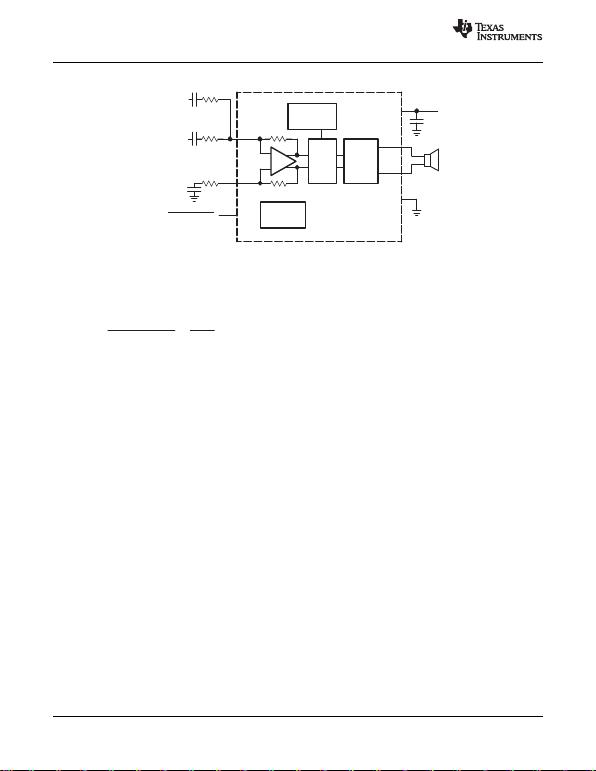_
+
IN–
IN+
PWM H–
Bridge
VO+
VO–
Internal
Oscillator CS
To Battery
VDD
GND
Bias
Circuitry
RI2
RP
Filter-Free Class D
SHUTDOWN
RI1
Single-Ended
Input 2
CI2
CP
Single-Ended
Input 1
CI1
qJA +1
Derating Factor +1
0.016 +62.5°CńW
TAMax +TJMax *qJAPDmax +150 *62.5 (0.2) +137.5°C
TPA2005D1-Q1
SLOS474C AUGUST 2005REVISED MARCH 2010
www.ti.com
Figure 31. Application Schematic With TPA2005D1 Summing Two Single-Ended Inputs
EFFICIENCY AND THERMAL INFORMATION
The maximum ambient temperature depends on the heat-sinking ability of the PCB system. The derating factor
for the 2,5-mm x 2,5-mm MicroStar Junior package is shown in the dissipation rating table. Converting this to qJA:
(17)
Given qJA of 62.5°C/W, the maximum allowable junction temperature of 150°C, and the maximum internal
dissipation of 0.2 W (worst case 5-V supply), the maximum ambient temperature can be calculated with equation
Equation 18.
(18)
Equation 18 shows that the calculated maximum ambient temperature is 137.5°C at maximum power dissipation
with a 5-V supply; however, the maximum ambient temperature of the package is limited to 85°C. Because of the
efficiency of the TPA2005D1, it can be operated under all conditions to an ambient temperature of 85°C. The
TPA2005D1 is designed with thermal protection that turns the device off when the junction temperature
surpasses 150°C to prevent damage to the IC. Also, using speakers more resistive than 8 dramatically
increases the thermal performance by reducing the output current and increasing the efficiency of the amplifier.
BOARD LAYOUT
Component Location
Place all the external components very close to the TPA2005D1. The input resistors need to be very close to the
TPA2005D1 input pins so noise does not couple on the high-impedance nodes between the input resistors and
the input amplifier of the TPA2005D1. Placing the decoupling capacitor, CS, close to the TPA2005D1 is important
for the efficiency of the class-D amplifier. Any resistance or inductance in the trace between the device and the
capacitor can cause a loss in efficiency.
Trace Width
Make the high current traces going to pins VDD, GND, VO+ and VO– of the TPA2005D1 have a minimum width of
0,7 mm. If these traces are too thin, the TPA2005D1 performance and output power will decrease. The input
traces do not need to be wide, but do need to run side-by-side to enable common-mode noise cancellation.
8-Pin QFN (DRB) Layout
Use the following land pattern for board layout with the 8-pin QFN (DRB) package. Note that the solder paste
should use a hatch pattern to fill solder paste at 50% to ensure that there is not too much solder paste under the
package.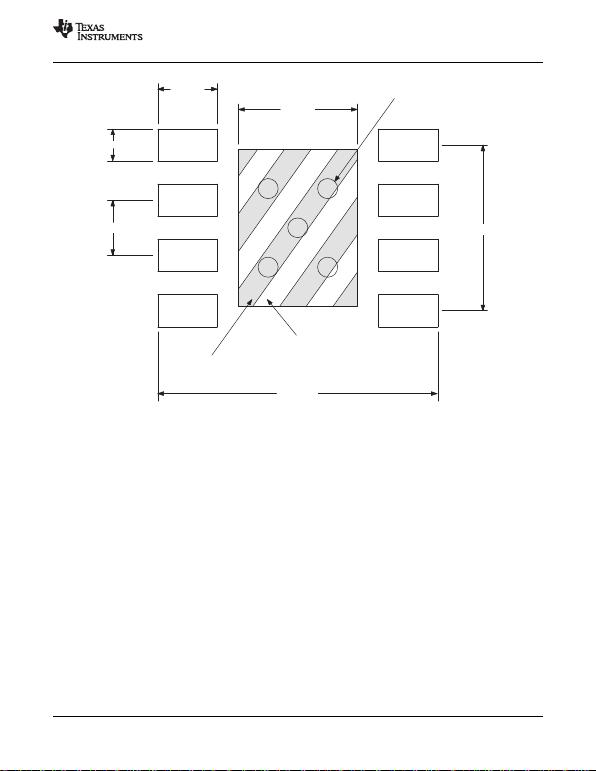0,65 mm
0,38 mm
Solder Mask: 1,4 mm x 1,85 mm centered in package
0,7 mm
1,4 mm
Make solder paste a hatch pattern to fill 50%
3,3 mm
1,95 mm
0,33 mm plugged vias (5 places)
TPA2005D1-Q1
www.ti.com
SLOS474C AUGUST 2005REVISED MARCH 2010
Figure 32. TPA2005D1 8-Pin QFN (DRB) Board Layout (Top View)
ELIMINATING THE OUTPUT FILTER WITH THE TPA2005D1
This section focuses on why the user can eliminate the output filter with the TPA2005D1.
Effect on Audio
The class-D amplifier outputs a pulse-width modulated (PWM) square wave, which is the sum of the switching
waveform and the amplified input audio signal. The human ear acts as a band-pass filter such that only the
frequencies between approximately 20 Hz and 20 kHz are passed. The switching frequency components are
much greater than 20 kHz, so the only signal heard is the amplified input audio signal.
The traditional class-D modulation scheme, which is used in the TPA005Dxx family, has a differential output in
which each output is 180 degrees out of phase and changes from ground to the supply voltage, VDD. Therefore,
the differential pre-filtered output varies between positive and negative VDD, where filtered 50% duty cycle yields
0 V across the load. The traditional class-D modulation scheme with voltage and current waveforms is shown in
Figure 33. Note that, even at an average of 0 V across the load (50% duty cycle), the current to the load is high,
causing a high loss and thus causing a high supply current.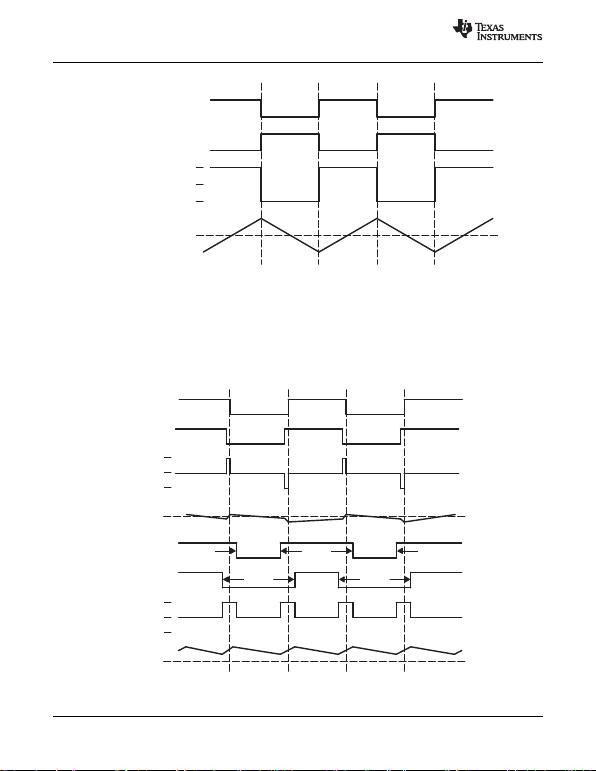0 V
–5 V
+5 V
Current
OUT+
Differential Voltage
OUT–
0 V
–5 V
+5 V
Current
OUT+
OUT–
Differential
Voltage
Across
0 V
–5 V
+5 V
Current
OUT+
OUT–
Differential
Voltage
Across
Output = 0 V
Output > 0 V
TPA2005D1-Q1
SLOS474C AUGUST 2005REVISED MARCH 2010
www.ti.com
Figure 33. Traditional Class-D Modulation Scheme Output Voltage and Current Waveforms Into an
TPA2005D1 Modulation Scheme
The TPA2005D1 uses a modulation scheme that still has each output switching from 0 to the supply voltage.
However, OUT+ and OUT– are now in phase with each other, with no input. The duty cycle of OUT+ is greater
than 50% and OUT– is less than 50% for positive voltages. The duty cycle of OUT+ is less than 50% and OUT–
is greater than 50% for negative voltages. The voltage across the load remains at 0 V throughout most of the
switching period, greatly reducing the switching current, which reduces any I2R losses in the load.
Figure 34. The TPA2005D1 Output Voltage and Current Waveforms Into an Inductive Load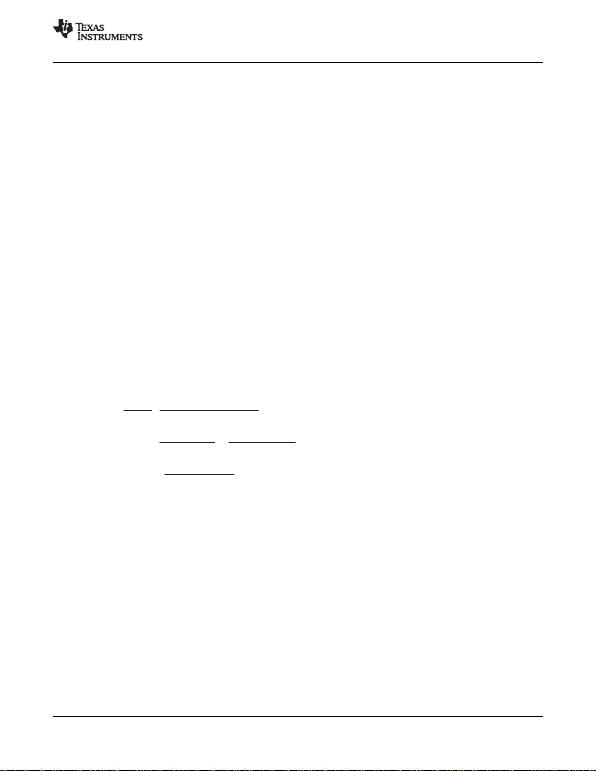PSPKR +PSUP–PSUP THEORETICAL (at max output power)
PSPKR +
PSUP
POUTPSUP THEORETICAL
POUT (at max output power)
PSPKR +POUT ǒ1
hMEASURED *1
hTHEORETICALǓ(at max output power)
hTHEORETICAL +RL
RL)2rDS(on) (at max output power)
TPA2005D1-Q1
www.ti.com
SLOS474C AUGUST 2005REVISED MARCH 2010
Efficiency: Why You Must Use a Filter With the Traditional Class-D Modulation Scheme
The main reason that the traditional class-D amplifier needs an output filter is that the switching waveform results
in maximum current flow. This causes more loss in the load, which causes lower efficiency. The ripple current is
large for the traditional modulation scheme because the ripple current is proportional to voltage multiplied by the
time at that voltage. The differential voltage swing is 2 × VDD, and the time at each voltage is one-half the period
for the traditional modulation scheme. An ideal LC filter is needed to store the ripple current from each half-cycle
for the next half-cycle, while any resistance causes power dissipation. The speaker is both resistive and reactive,
whereas an LC filter is almost purely reactive.
The TPA2005D1 modulation scheme has very little loss in the load without a filter because the pulses are very
short and the change in voltage is VDD instead of 2 × VDD. As the output power increases, the pulses widen,
making the ripple current larger. Ripple current could be filtered with an LC filter for increased efficiency, but for
most applications the filter is not needed.
An LC filter with a cutoff frequency less than the class-D switching frequency allows the switching current to flow
through the filter instead of the load. The filter has less resistance than the speaker, resulting in less power
dissipation, which increases efficiency.
Effects of Applying a Square Wave Into a Speaker
If the amplitude of a square wave is high enough and the frequency of the square wave is within the bandwidth
of the speaker, a square wave could cause the voice coil to jump out of the air gap and/or scar the voice coil. A
250-kHz switching frequency, however, is not significant because the speaker cone movement is proportional to
1/f2for frequencies beyond the audio band. Therefore, the amount of cone movement at the switching frequency
is very small. However, damage could occur to the speaker if the voice coil is not designed to handle the
additional power. To size the speaker for added power, the ripple current dissipated in the load must be
calculated by subtracting the theoretical supplied power, PSUP THEORETICAL, from the actual supply power, PSUP, at
maximum output power, POUT. The switching power dissipated in the speaker is the inverse of the measured
efficiency,hMEASURED, minus the theoretical efficiency,hTHEORETICAL.
(19)
(20)
(21)
(22)
The maximum efficiency of the TPA2005D1 with a 3.6-V supply and an 8-load is 86% from Equation 22. Using
Equation 21 with the efficiency at maximum power (84%), we see that there is an additional 17 mW dissipated in
the speaker. The added power dissipated in the speaker is not an issue as long as it is taken into account when
choosing the speaker.
When to Use an Output Filter
Design the TPA2005D1 without an output filter if the traces from amplifier to speaker are short. The TPA2005D1
passed FCC and CE radiated emissions with no shielding and with speaker trace wires 100 mm long or less.
Wireless handsets and PDAs are great applications for class-D without a filter.
A ferrite bead filter often can be used if the design is failing radiated emissions without an LC filter, and the
frequency-sensitive circuit is greater than 1 MHz. This is good for circuits that just have to pass FCC and CE
because FCC and CE only test radiated emissions greater than 30 MHz. If choosing a ferrite bead, choose one
with high impedance at high frequencies, but very low impedance at low frequencies.
Use an LC output filter if there are low-frequency (<1 MHz) EMI-sensitive circuits and/or there are long leads
from amplifier to speaker.
Figure 35 and Figure 36 show typical ferrite bead and LC output filters.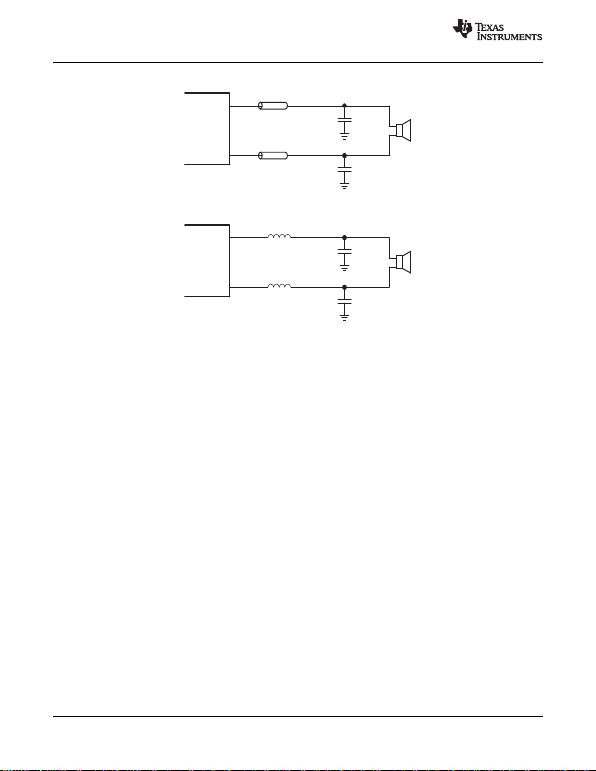1 nF
Ferrite
OUTP
OUTN
Ferrite
1 nF
1 µF
1 µF
33 µH
33 µH
OUTP
OUTN
TPA2005D1-Q1
SLOS474C AUGUST 2005REVISED MARCH 2010
www.ti.com
Figure 35. Typical Ferrite Chip Bead Filter (Chip bead example: NEC/Tokin: N2012ZPS121)
Figure 36. Typical LC Output Filter, Cut-Off Frequency of 27 kHz